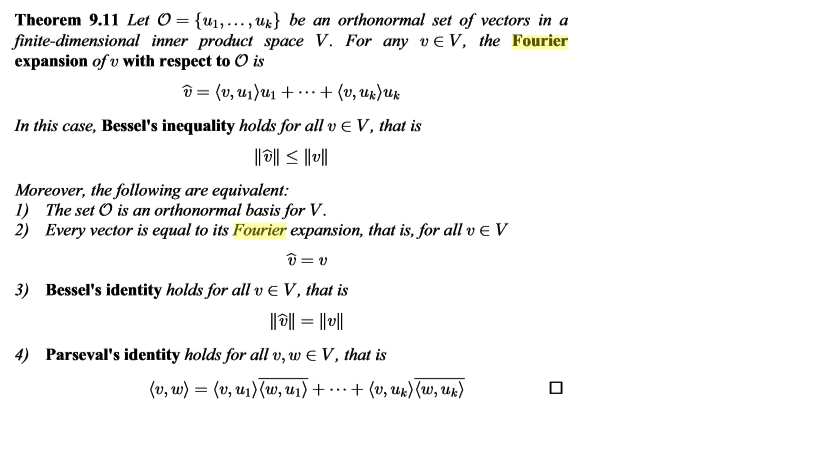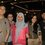# Prove it equivalentHelp me please provet it 1,2,3,and 4 are equivalentNote by Ahmad Nugroho
2 years, 7 months ago

MarkdownAppears as
*italics* or _italics_ italics
**bold** or __bold__ bold
- bulleted- list
• bulleted
• list
1. numbered2. list
1. numbered
2. list
Note: you must add a full line of space before and after lists for them to show up correctly
paragraph 1paragraph 2

paragraph 1

paragraph 2

[example link](https://brilliant.org)example link
> This is a quote
This is a quote
    # I indented these lines
# 4 spaces, and now they show
# up as a code block.

print "hello world"
# I indented these lines
# 4 spaces, and now they show
# up as a code block.

print "hello world"
MathAppears as
Remember to wrap math in $$...$$ or $...$ to ensure proper formatting.
2 \times 3 $$2 \times 3$$
2^{34} $$2^{34}$$
a_{i-1} $$a_{i-1}$$
\frac{2}{3} $$\frac{2}{3}$$
\sqrt{2} $$\sqrt{2}$$
\sum_{i=1}^3 $$\sum_{i=1}^3$$
\sin \theta $$\sin \theta$$
\boxed{123} $$\boxed{123}$$

## Comments

Sort by:

Top Newest

What have you done? What have you tried? Where are you stuck?

Staff - 2 years, 7 months ago

Log in to reply

From point 4 to point 1 i stucked no idea

- 2 years, 7 months ago

Log in to reply

How do you show that a set is an orthonormal basis? What are the necessary and sufficient conditions?

Staff - 2 years, 7 months ago

Log in to reply

If we have an orthornormal set like the picture , the condition for orthonormal basis are <ui,uj> = 0 , u~=j <ui,uj> = 1 , u=j

<,> is an inner product Sorry i replied by phone

- 2 years, 7 months ago

Log in to reply

×

Problem Loading...

Note Loading...

Set Loading...